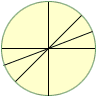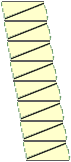I am doing a maths assigment for university, which is aimed towrds primary school students(k-6). I was wondering if you could give me some information as to how I could describe to students the rule for finding the area of circle, using a circle cut up into equal sectors (like a pizza). I know it has something to do with the fact that you can make these shapes into a parallelogram, but I am a bit uncertain as to how I can express this idea clearly and articulately to students. Thanks for your help Jessica Hi Jessica, Cut the circle into segments as in the diagram below, in this case 16 segments. Stack the segments to form a shape which is approximately a parallelogram.The length of the base of the parallelogram is the radius of the circle and the height of the parallelogram is approximately half the circumference of the circle. Since the circumference of a circle is two pi times its radius, the area of the parallelogram is approximately pi times the square of the radius of the circle. Cheers, Penny Go to Math Central